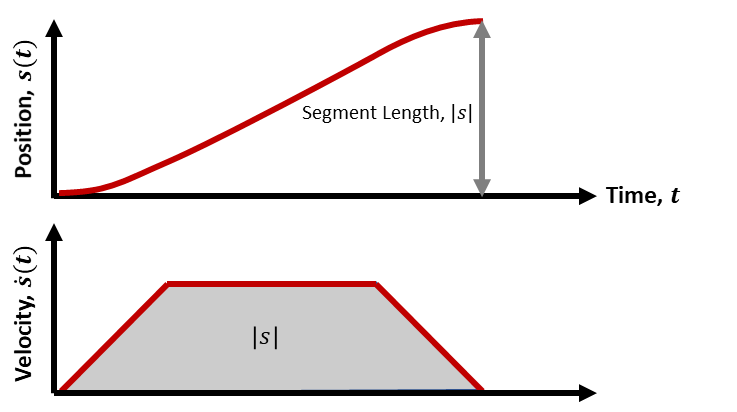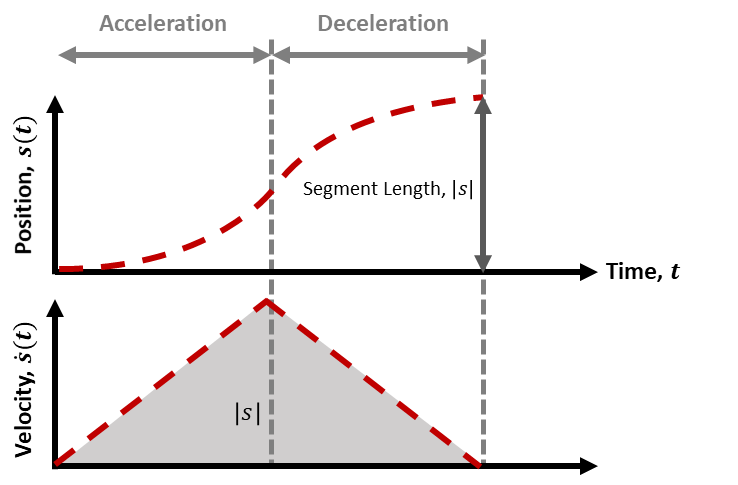# Design Trajectory with Velocity Limits Using Trapezoidal Velocity Profile

This example shows how to use the trapezoidal velocity profile to design a trajectory with input bounds rather than parameters.

The `trapveltraj` function creates trajectories with trapezoidal velocity profiles. These trajectories follow a three-segment path of acceleration, constant velocity, and deceleration between all of their waypoints. You can alter these trajectories by specifying parameters to construct your desired profile, but there are many applications that require a set of bounds instead, such as limits on acceleration or velocity. In such applications, you must first translate these bounds into a parameterized trajectory that is both feasible and satisfies the expected bounds. In this example, use the `helperProfileForMaxVel` helper function to create feasible trapezoidal profiles given a velocity bound.

### Basic `trapveltraj` Usage

If only the acceleration, constant velocity, and deceleration are known, you can create a trapezoidal velocity profile trajectory by interpolating the waypoints along each dimension using the specified parameters.

```% Define a set of 2-D waypoints and connect them using a trapezoidal % profile where each segment has a duration of 1 second wpts = [-1 1 .3; 1 1 -1]; [q,qd,qdd,t] = trapveltraj(wpts,100,EndTime=1);```

Visualize the results by using the `helperPlotTaskSpaceTraj` helper function, which creates a figure that plots the 2-D trajectory on the left and the position and velocity with respect to time on the right. Since all of the end times match, this trajectory hits both its vertical and horizontal waypoints simultaneously, making it suitable for numerous applications.

```tpts = 0:size(wpts,2)-1; helperPlotTaskSpaceTraj("EndTime = 1",t,q,qd,wpts,tpts);```### Determine Limitations Using Profile Parameters as Bounds

Try to create a trajectory for a two-degree-of-freedom (2-DoF) robot where the maximum joint velocity is 0.5 rad/s, using the same waypoints. Specify the peak velocity of the trajectory as `0.5`. This sets a specified velocity to achieve in each segment of the trajectory, and because the distances between waypoints can vary across dimensions, each dimension may have a different segment length. This can create unintended results.

Visualize the results. The generated trajectory is acceptable if the AI must only connect the first and last waypoints in time, but the different segment lengths along dimensions, can make it insufficient for additional tasks, such as collision avoidance, or if using the trajectory to meet points in 3-D space. If the trajectory must meet every waypoint, you can improve it by specifying the end time. This ensures that each segment has the same length.

```[q,qd,qdd,t] = trapveltraj(wpts,100,PeakVelocity=0.5); helperPlotTaskSpaceTraj("PeakVelocity = 0.5 rad/s",t,q,qd,wpts);```### Trapezoidal Velocity Profile Overview

The trapezoidal velocity profile trajectory connects waypoints using a motion profile that stops at each waypoint, and where the waypoint-to-waypoint motion is governed by the following motion profile:As shown in the image above, the velocity profile has four parameters:

• End Time — Duration of each segment between two waypoints

• Peak Velocity Peak velocity for a each segment

• Acceleration Time — Time spent in the acceleration and deceleration phases of each segment

• Peak Acceleration — Magnitude of the acceleration applied during the acceleration and deceleration phases of each segment

You can fully define a profile by specifying two of these. When you provide only one parameter, `trapveltraj` automatically assigns a second parameter so that the average velocity of each segment is halfway between the two allowable bounds detailed in Constraints for Combining Parameter Specifications.

Mathematically, this profile defines segments on the interval `[0, endTime]`, where each segment has an acceleration phase, constant speed phase, and a deceleration phase. The lengths of the acceleration and deceleration phases, `accelTime`, are equal.

1. Acceleration Phase — For $\mathit{t}$ = [0, `accelTime`], $\frac{{\mathrm{d}}^{2}}{\mathrm{d}{\mathit{t}}^{2}}\mathit{s}\left(\mathit{t}\right)=\mathit{a},\text{\hspace{0.17em}}\frac{d}{\mathrm{d}\mathit{t}}\mathit{s}\left(\mathit{t}\right)=\mathit{at},\text{\hspace{0.17em}}\mathit{s}\left(\mathit{t}\right)=\mathit{a}\frac{{\mathit{t}}^{2}}{2}$

2. Constant Speed Phase For $\mathit{t}$ = [`accelTime`, `endTime``accelTime`], $\frac{{\mathrm{d}}^{2}}{\mathrm{d}{\mathit{t}}^{2}}\mathit{s}\left(\mathit{t}\right)=0,\text{\hspace{0.17em}}\frac{d}{\mathrm{d}\mathit{t}}\mathit{s}\left(\mathit{t}\right)=\mathit{v},\text{\hspace{0.17em}}\mathit{s}\left(\mathit{t}\right)=\mathit{v}\mathit{t}-\frac{{\mathit{v}}^{2}}{2\mathit{a}}$

3. Deceleration Phase For $\mathit{t}$ = [`endTime``accelTime`, `endTime`], $\frac{{\mathrm{d}}^{2}}{\mathrm{d}{\mathit{t}}^{2}}\mathit{s}\left(\mathit{t}\right)=-\mathit{a},\text{\hspace{0.17em}}\frac{d}{\mathrm{d}\mathit{t}}\mathit{s}\left(\mathit{t}\right)=\mathit{a}\left(\mathrm{endTime}-\mathit{t}\right),\text{\hspace{0.17em}}\mathit{s}\left(\mathit{t}\right)=\frac{2\mathit{av}*\mathrm{endTime}-2{\mathit{v}}^{2}-{{\mathit{a}}^{2}\left(t-\mathrm{endTime}\right)}^{2}}{\left(2a\right)}$

### Constraints for Combining Parameter Specifications

The profile design results in two limiting constraints. The magnitude of the peak velocity must be:

• Peak velocity must be greater than the average speed: $\mathit{peakVelocity}>\frac{\mathit{s}\left(\mathrm{endTime}\right)-\mathit{s}\left(0\right)}{\mathrm{endTime}}$

• Peak velocity must be less than or equal to twice the average speed:$\text{\hspace{0.17em}}\mathit{peakVelocity}\underset{‾}{<}2\left(\frac{\mathit{s}\left(\mathrm{endTime}\right)-\mathit{s}\left(0\right)}{\mathrm{endTime}}\right)$

These constraints are derived from the total segment length $|\mathit{s}|=|\mathit{s}\left(\mathrm{End}\text{\hspace{0.17em}}\mathrm{Time}\right)-\mathit{s}\left(0\right)|$, which is fixed with any two waypoints and results from the integral of the velocity, i.e. the area of the trapezoid:The first constraint sets the lowest allowable peak velocity magnitude. This occurs when the motion spends the most amount of time at constant speed:In the second case, the acceleration and deceleration phases are infinitesimal, resulting in a nearly rectangular velocity profile that has a total area $\mathit{A}=\left(\mathrm{Width}*\mathrm{Length}\right)$, or $|\mathit{s}|=|\mathrm{peakVelocity}|*\left(\mathrm{endTime}\right)$. Hence for this case to succeed, the magnitude of the peak velocity must equal the average speed $\frac{|\mathit{s}|}{\mathrm{endTime}}$. In practice, the acceleration / deceleration phases can never be truly infinitesimal (Acceleration Time > 0), which instead makes this an inequality constraint: $\mathrm{peakVelocity}>\frac{|\mathit{s}|}{\mathrm{endTime}}$.

The second constraint defines the highest allowable peak velocity. This occurs when the motion spends as much time as possible accelerating to a peak velocity:In this situation, there is no constant speed phase, resulting in a triangular velocity profile, which has a total area $\mathit{A}=\frac{\mathrm{Height}*\mathrm{Length}}{2}$ or $|\mathit{s}|=\frac{|\mathrm{Peak}\text{\hspace{0.17em}}\mathrm{Velocity}|*\left(\mathrm{End}\text{\hspace{0.17em}}\mathrm{Time}\right)}{2}$. Therefore, in this extreme, the magnitude of the peak velocity must equal twice the peak speed, resulting in the second constraint, $|\mathrm{Peak}\text{\hspace{0.17em}}\mathrm{Velocity}|>\frac{2|\mathit{s}|}{\mathrm{endTime}}$.

These two constraints define the viable range of trapezoidal velocity profiles for a given segment. The following graphic compares three such trajectories, with the first constraint shown using dotted lines, the second shown with dashed lines, and an example of a trapezoidal profile within these constraints shown as a solid line. All plausible trapezoidal profile trajectories for this segment must fall between the dotted and dashed lines, as some variation of the solid line example.### Limitations on Parameter Combinations

In general, any two of the four defining parameters (End Time, Peak Velocity, Peak Acceleration, Acceleration Time) can be used together, but you must ensure that it is possible to generate feasible trajectories within the limits of the profile. For example, suppose there is a segment between point 0 and 1 rad, and the desired segment duration is 1 second.

```[q,qd]=trapveltraj([0 1],100,'EndTime',1,'PeakVelocity',1); ```

Given the constraints derived above, for the segment from s = 0 to s = 1, the constraints imply that: $|\mathrm{Peak}\text{\hspace{0.17em}}\mathrm{Velocity}|\left(\mathrm{End}\text{\hspace{0.17em}}\mathrm{Time}\right)>1$ and $|\mathrm{Peak}\text{\hspace{0.17em}}\mathrm{Velocity}|\left(\mathrm{End}\text{\hspace{0.17em}}\mathrm{Time}\right)<2$, whereas with the values used above, the first condition is not met. This could be resolved e.g. by raising the peak velocity to 1.5.

Limitations can also arise because the constraints work for one dimension of a segment, but not for all of them. For example, suppose there is a segment between [0 0] and [1 2], with assigned values for the segment duration and peak velocity:

```[q,qd]=trapveltraj([0 1; 0 2],100,'EndTime',1,'PeakVelocity',1.5); ```

This will fail because the constraints only work for the first dimension:

• For the first dimension, from s = 0 to s = 1, the constraints imply that: $|\mathrm{Peak}\text{\hspace{0.17em}}\mathrm{Velocity}|\left(\mathrm{End}\text{\hspace{0.17em}}\mathrm{Time}\right)>1$ and $|\mathrm{Peak}\text{\hspace{0.17em}}\mathrm{Velocity}|\left(\mathrm{End}\text{\hspace{0.17em}}\mathrm{Time}\right)<2$

• For the second dimension, from s= 0 to s = 2, the constraints imply that: $|\mathrm{Peak}\text{\hspace{0.17em}}\mathrm{Velocity}|\left(\mathrm{End}\text{\hspace{0.17em}}\mathrm{Time}\right)>2$ and $|\mathrm{Peak}\text{\hspace{0.17em}}\mathrm{Velocity}|\left(\mathrm{End}\text{\hspace{0.17em}}\mathrm{Time}\right)<4$

It is evident that these constraints cannot be met if the same values are used for both dimensions. Instead, it is necessary to use different peak velocities for each dimension to ensure that the waypoints can be hit with the same end times. For example, the following solution works:

```wpts2 = [0 1; 0 2]; [q,qd,qdd,t]=trapveltraj(wpts2,100,'EndTime',1,'PeakVelocity',[1.5; 2.5]); helperPlotTaskSpaceTraj('Different Peak Velocities for each Dimension',t,q,qd,wpts2);```Choosing parameters that satisfy all constraints along each dimension can be challenging. In the next section, a helper function is instead created to help simplify this process.

### Create a Helper Function to Translate Velocity Bounds to Profile Parameters

The `helperProfileForMaxVel` helper function accepts a limiting segment velocity and outputs a set of end times and velocities that ensure all dimensions have the same segment length, and that the maximum velocity of any segment is less than or equal to the specified velocity maximum.

```function [endTimes,peakVels] = helperProfileForMaxVel(wpts,maxVelocity) % helperProfileForMaxVel Generate parameters for a trapezoidal velocity profile so it will meet velocity constraints % % This function creates a fast trapezoidal profile with a specified velocity limit as an upper bound. % Copyright 2021 The MathWorks, Inc. % Check that maxVelocity is a scalar validateattributes(maxVelocity,{'numeric'},{'scalar'}); % Find segment lengths along each dimension of waypoints. segLengths = abs(diff(wpts,1,2)); % Find minimum endTime by assigning maximum velocity to longest segment maxSegLengths = max(segLengths,[],1); endTimeLowerBound = (maxSegLengths/maxVelocity); % Choose acceleration by multiplying endTime to be greater than lower bound greaterThanFactor = 1.1; endTimes = repmat(greaterThanFactor*endTimeLowerBound,size(wpts,1),1); % Determine min and max peak velocity for each segment and dimension % Choose largest velocity that doesn't exceed maximum velocity minPeakVels = segLengths./endTimes; maxPeakVels = 2*segLengths./endTimes; peakVels = min(maxPeakVels,maxVelocity); peakVels = max(minPeakVels,peakVels); % Replace any zero-values with peak velocity peakVels(peakVels==0) = maxVelocity; end ```

Use the helper function to create a trajectory for the waypoints that has a maximum velocity bound of 0.5 rad/s.

```[endTimes,peakVels] = helperProfileForMaxVel(wpts,0.5); [q,qd,qdd,t,pp] = trapveltraj(wpts,100,EndTime=endTimes,PeakVelocity=peakVels); tpts = [0 cumsum(endTimes(1,:))]; helperPlotTaskSpaceTraj("Helper Function with Maximum Velocity 0.5 rad/s",t,q,qd,wpts,tpts);```### References

 Lynch, Kevin M., and Frank C. Park. Modern Robotics: Mechanics, Planning and Control. Cambridge: Cambridge University Press, 2017.